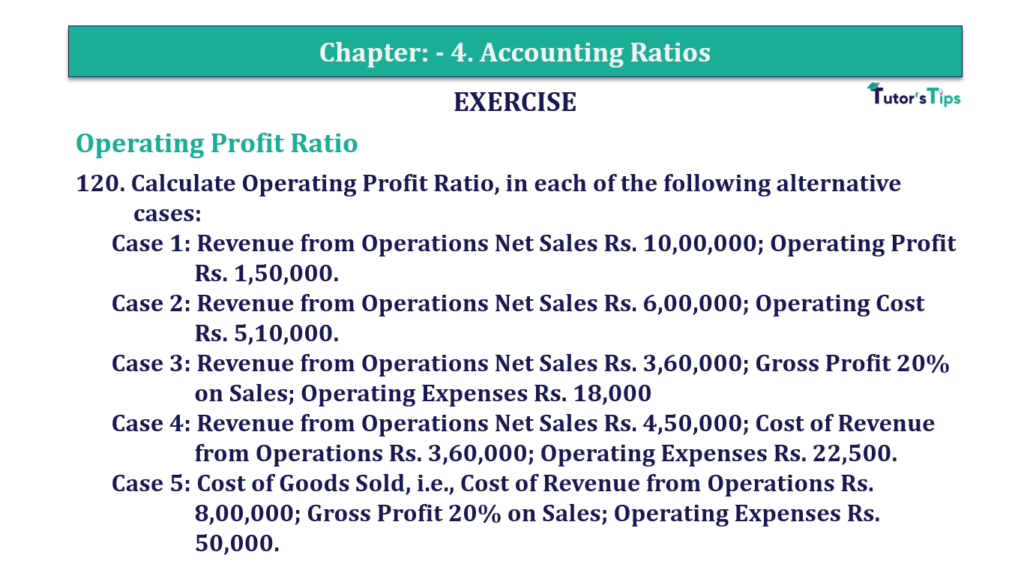# Question 120 Chapter 4 of +2-B – T.S. Grewal 12 ClassQuestion 120 Chapter 4 of +2-B

Operating Ratio

120. Calculate Operating Profit Ratio, in each of the following alternative
cases:
Case 1: Revenue from Operations Net Sales Rs. 10,00,000; Operating Profit
Rs. 1,50,000.
Case 2: Revenue from Operations Net Sales Rs. 6,00,000; Operating Cost
Rs. 5,10,000.
Case 3: Revenue from Operations Net Sales Rs. 3,60,000; Gross Profit 20%
on Sales; Operating Expenses Rs. 18,000
Case 4: Revenue from Operations Net Sales Rs. 4,50,000; Cost of Revenue
from Operations Rs. 3,60,000; Operating Expenses Rs. 22,500.
Case 5: Cost of Goods Sold, i.e., Cost of Revenue from Operations Rs.
8,00,000; Gross Profit 20% on Sales; Operating Expenses Rs.
50,000.

### The solution of Question 120 Chapter 4 of +2-B: –

Case I:

 Net Sales = Rs. 10,00,000 Operating Profit = Rs. 1,50,000

 Operating Ratio = Operating Cost X 100 Net Sales
 Operating Ratio = Rs. 1,50,000 X 100 Rs. 10,00,000 = 15%

Case II:

 Net Sales = Rs. 6,00,000 Operating Cost = Rs. 5,10,000 Operating Profit = Net Sales – Operating Cost = Rs. 6,00,000 – Rs. 5,10,000 = Rs. 90,000
 Operating Ratio = Operating Cost X 100 Net Sales
 Operating Ratio = Rs. 90,000 X 100 Rs. 6,00,000 = 15%

Case III:

 Net Sales = Rs. 3,60,000 Gross Profit = 20% on Sales
 Gross Profit = 20 X Rs.3,60,000 100 = Rs. 72,000
 Operating Profit = Gross Profit – Operating Cost = Rs. 72,000 – Rs. 18,000 = Rs. 54,000
 Operating Ratio = Operating Cost X 100 Net Sales
 Operating Ratio = Rs. 54,000 X 100 Rs. 3,60,000 = 15%

Case IV:

 Net Sales = Rs. 4,50,000 Cost of Goods Sold = Rs. 3,60,000 Operating Expenses = Rs. 3,60,000 Operating Profit = Gross Profit – Operating Cost = Rs. 4,50,000 – Rs. 3,60,000 – Rs. 22,500 = Rs. 67,500
 Operating Ratio = Operating Cost X 100 Net Sales
 Operating Ratio = Rs. 67,500 X 100 Rs. 4,50,000 = 15%

Case V:

 Gross Profit = 20% on Sales Sales = X
 Gross Profit = 20 X x 100
 Gross Profit = 20x 100
 Sales = Cost Goods Sold + Gross Profit
 X = 20x + Rs.8,00,000 100
 100x = 20x + Rs. 8,00,00,000 80x = Rs. 8,00,00,000
 Gross Profit = Rs. 8,00,00,000 80
 Operating Cost = Cost of Goods Sold + Operating Expenses = Rs. 8,00,000 + Rs. 50,0000 = Rs. 8,50,000 Operating Profit = Net Sales – Cost of Goods Sold – Operating Cost = Rs. 10,00,000 – Rs. 8,00,000 – Rs. 50,000 = Rs. 1,50,000
 Operating Ratio = Operating Cost X 100 Net Sales
 Operating Ratio = Rs. 1,50,000 X 100 Rs. 10,00,000 = 15%

Balance Sheet: Meaning, Format & Examples

Comment if you have any question.

Also, Check out the solved question of previous Chapters: –

### T.S. Grewal’s Double Entry Book Keeping +2 (Vol. I: Accounting for Not-for-Profit Organizations and Partnership Firms)

• Chapter No. 1 – Financial Statement of Not-For-Profit Organisations
• Chapter No. 2 – Accounting for Partnership Firms – Fundamentals
• Chapter No. 3 – Goodwill: Nature and Valuation
• Chapter No. 4 – Change in Profit-Sharing Ratio Among the Existing Partners
• Chapter No. 5 – Admission of a Partner
• Chapter No. 6 – Retirement/Death of a Partner
• Chapter No. 7 – Dissolution of a Partnership Firm

### T.S. Grewal’s Double Entry Book Keeping (Vol. II: Accounting for Companies)

• Chapter No. 1 – Financial Statements of a Company
• Chapter No. 2 – Financial Statement Analysis
• Chapter No. 3 – Tools of Financial Statement Analysis – Comparative Statements and Common- Size Statements
• Chapter No. 4 – Accounting Ratios
• Chapter No. 5 – Cash Flow Statement